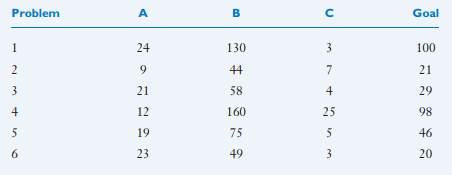Mental Set

Try these two examples to see the effects of mental set

A. Luchins’s Water-Jar Problem. Suppose that you have three jars, A, B, and C. Each of six problems (listed below) shows the capacity of the three jars. You must use these jars in order to obtain the amount of liquid specified in the Goal column. You may obtain the goal amount by adding or subtracting the quantities listed in A, B, and C. (The answers can be found later in the text, in the discussion of mental set.)B. A Number Puzzle. You are no doubt familiar with the kind of number puzzles in which you try to figure out the pattern for the order of numbers. Why are these numbers arranged in this order?

8, 5, 4, 9, 1, 7, 6, 3, 2, 0

The answer appears at the end of the chapter